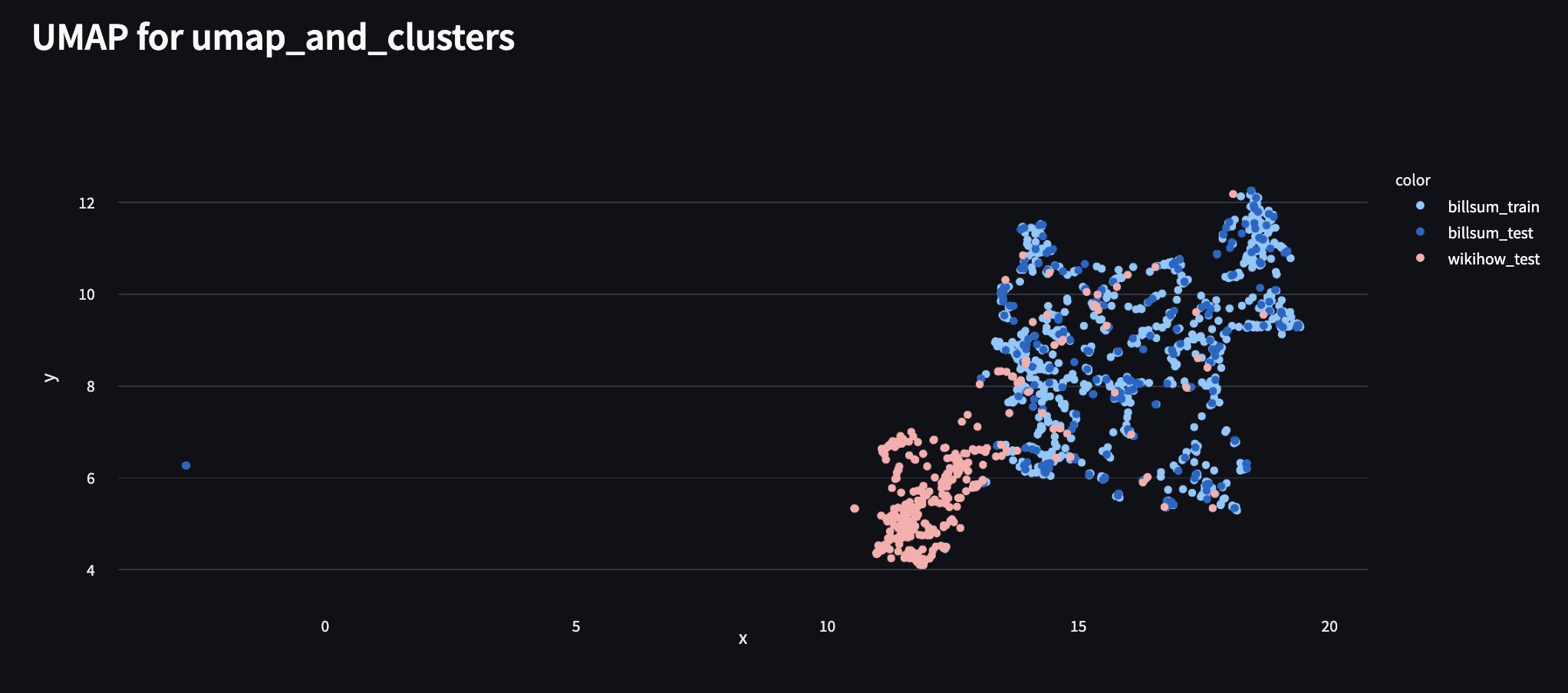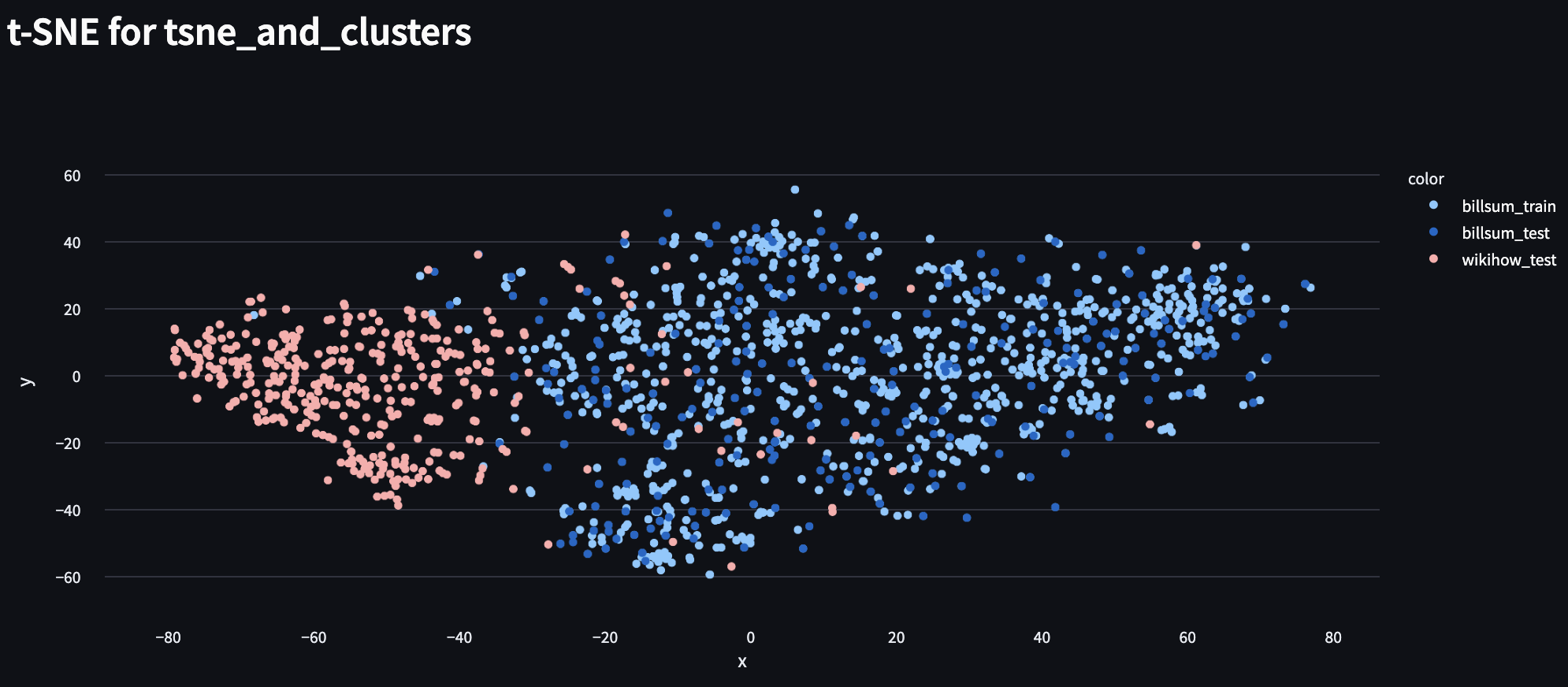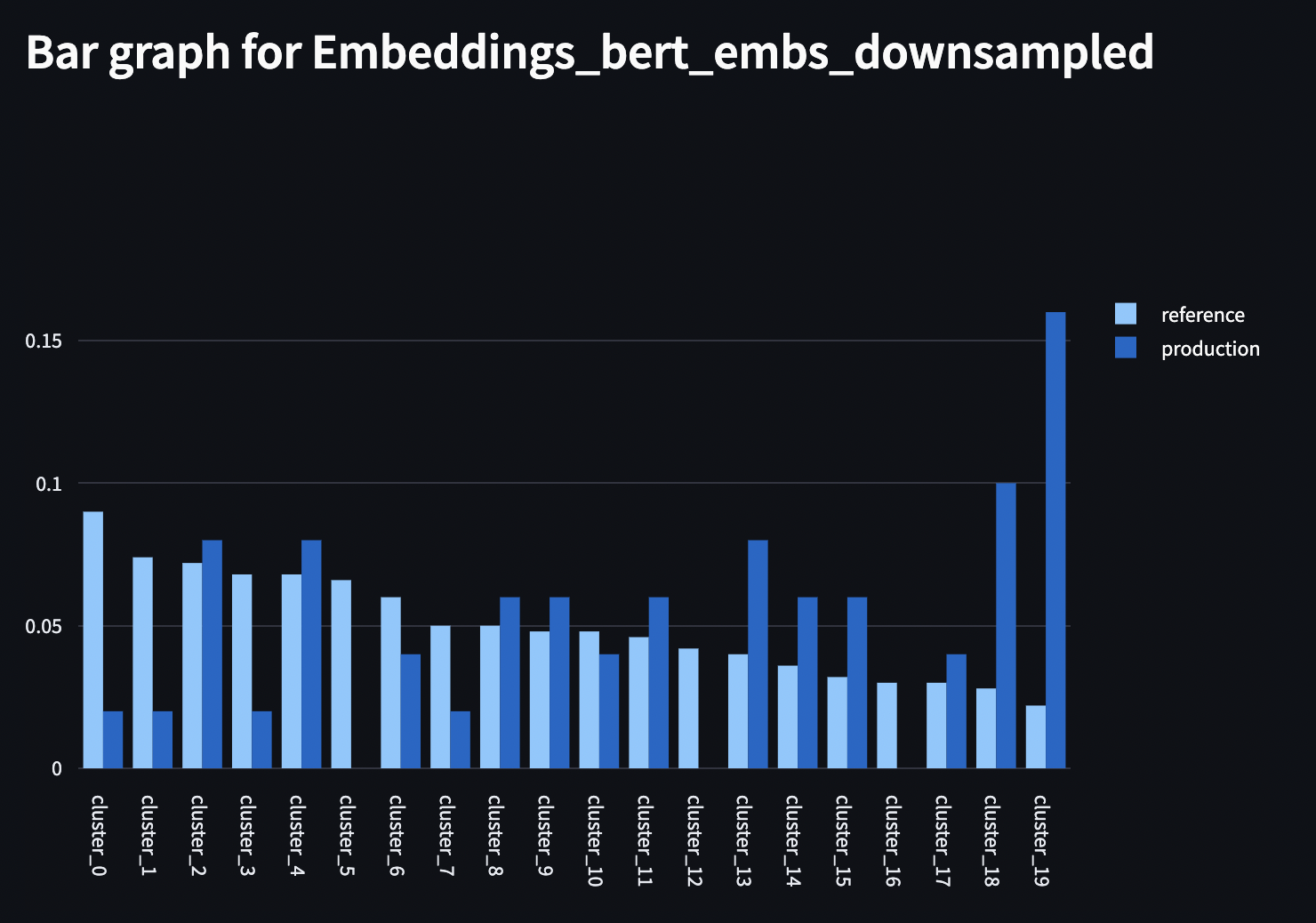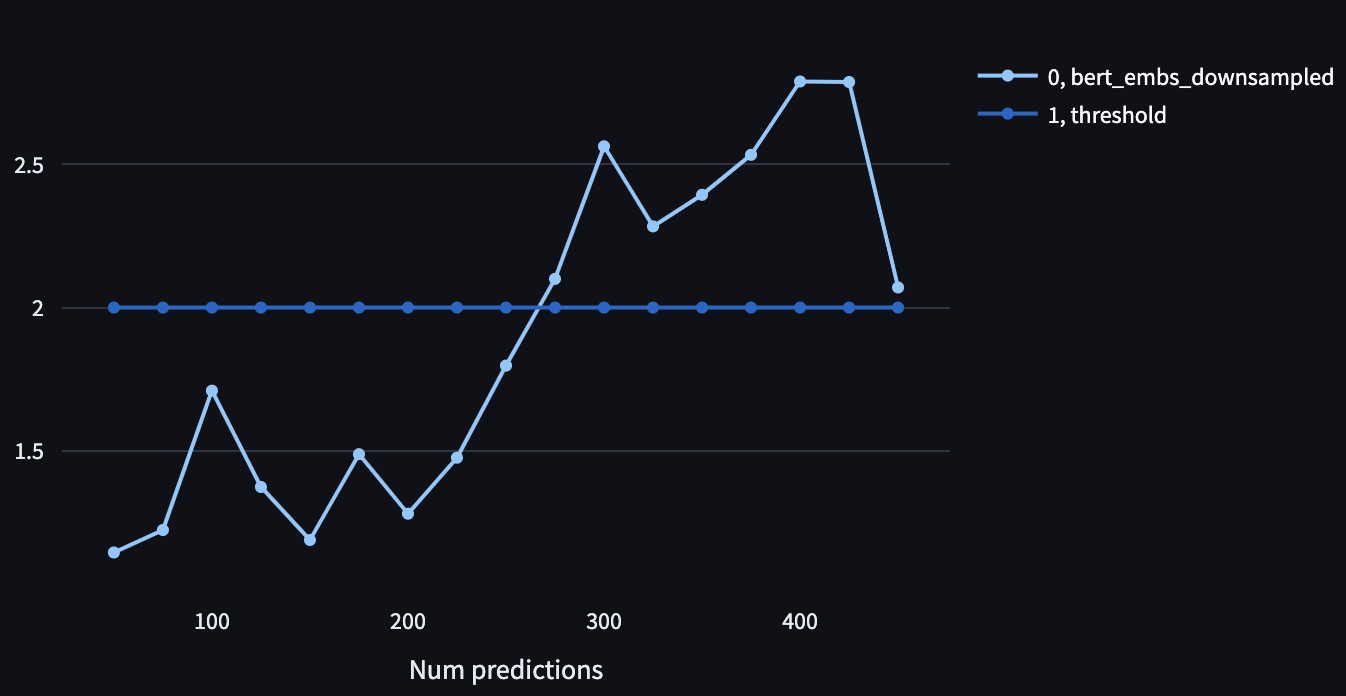Overview: In this example, we will see how to use UpTrain to monitor performance of a text summarization task in NLP. Summarization creates a shorter version of a document or an article that captures all the important information. For the same, we will be using a pretrained text summarization model (with T5 architecture) from Huggingface. This model was trained on the billsum dataset.

Why is monitoring needed: Monitoring NLP tasks with traditional metrics (such as accuracy) in production is hard, as groud truth is unavailable (or extremely delayed when there is a human in the loop). And, hence, it becomes very important to develop techniques to monitor real time monitoring for tasks such as text summarization before important business metrics (such as customer satisfaction and revenue) are affected.

Problem: In this example, the model was trained on the billsum dataset. This dataset contains the articles and their summarization of the US Congressional and California state bills. However, in production, we append some samples from the wikihow dataset. The WikiHow is a large-scale dataset using the online WikiHow knowledge base. As you can imagine, the two datasets are quite different. It would be interesting to see how the text summarization task performs in production 🤔

Solution: We will be using UpTrain framework which provides an easy-to-configure way to log training data, production data and model’s predictions. We apply several techniques on theis logged data, such as clustering, data drift detection and customized signals, to monitor performance and raise alerts in case of any dip in model’s performance 🚀

## ​Step 1: Setup - Defining model and datasets

### ​Define model and tokenizer for the summarization task

``````tokenizer_t5 = AutoTokenizer.from_pretrained("t5-small")
model_t5 = AutoModelForSeq2SeqLM.from_pretrained("t5-small")
prefix = "summarize: "
``````

### ​Load Billsum dataset from Huggingface which was used to train our model

``````billsum_dataset = load_dataset("billsum", split="ca_test").filter(lambda x: x['text'] is not None)
billsum = billsum_dataset.train_test_split(test_size=0.2)
billsum
``````
``````DatasetDict({
train: Dataset({
features: ['text', 'summary', 'title'],
num_rows: 989
})
test: Dataset({
features: ['text', 'summary', 'title'],
num_rows: 248
})
})
``````

Create a small test dataset from the Wikihow dataset to test our summarization model. Download the wikihow dataset from https://ucsb.app.box.com/s/ap23l8gafpezf4tq3wapr6u8241zz358 and save it as ‘wikihowAll.csv’ in the current directory.

``````download_wikihow_csv_file()
wikihow_dataset = load_dataset("csv", data_files='wikihowAll.csv').filter(lambda x: x['text'] is not None)
wikihow = wikihow_dataset['train'].train_test_split(test_size=453)
wikihow
``````
``````    DatasetDict({
train: Dataset({
features: ['summary', 'title', 'text'],
num_rows: 213841
})
test: Dataset({
features: ['summary', 'title', 'text'],
num_rows: 453
})
})
``````

### ​Create a test dataset by combining billsum and wikihow datasets

``````final_test_dataset = combine_datasets(billsum["test"], 'billsum_test', wikihow['test'], 'wikihow_test')
final_test_dataset
``````
``````Dataset({
features: ['text', 'summary', 'title', 'dataset_label'],
num_rows: 701
})
``````

### ​Let’s try out our model on one of the sample

``````sample_text = final_test_dataset.filter(lambda x: len(x["text"]) < 1000)['text']
input_embs = tokenizer_t5(prefix + sample_text, truncation=True, padding=True, return_tensors="pt").input_ids
summary = tokenizer_t5.batch_decode(model_t5.generate(input_embs), skip_special_tokens=True)
print({"model_input_text_to_summarize": sample_text}, "\n")
print({"model_output_summary": summary})
``````
``````{'model_input_text_to_summarize': ' Bring the rice to a boil, and then reduce the heat to a simmer for about 20 minutes while covered.;\n, Stir well and fluff the rice with a fork.\n\n,, Remove the stem, veins, and seeds from your jalapeño pepper. Slice it into strips using a cutting knife. Set aside.\n\n, Peel and seed the cucumber, and then slice it into strips with a cutting knife. Slice the avocado into small slices as well. Set aside.\n\n, The ropes should be long enough to spread on the seaweed sheets., Cover it with a sheet of nori.,, Repeat again with another seaweed sheet.,,, Place the sushi on a serving plate. Sprinkle over the seeds and dump into the sauce if desired. Enjoy!\n\n'}

{'model_output_summary': ['bring the rice to a boil, and then reduce the heat to a simmer for about']}
``````

## ​Using embeddings for model monitoring

To compare the two datasets, we will be utilizing text embeddings (generated by BERT). As we will see below, we can see clear differentiations between the two datasets in the embeddings space which could be an important metric to track drifts

#### ​Save bert embeddings for the training data

``````data_with_embs = generate_reference_dataset_with_embeddings(billsum['train'], tokenizer_t5, model_t5, dataset_label="billsum_train")
data_with_embs.keys()
``````
``````dict_keys(['id', 'dataset_label', 'title', 'text', 'model_output', 'bert_embs', 'bert_embs_downsampled'])
``````

## ​Step 2: Visualizing embeddings using UpTrain

Let’s first visualize how does the embeddings of the training dataset compares against that of our real-world testing dataset. We use two dimensionality reduction techniques, UMAP and t-SNE, for embedding visualization.

``````config = {
"checks": [
{
'type': uptrain.Visual.UMAP,
"measurable_args": {
'type': uptrain.MeasurableType.INPUT_FEATURE,
'feature_name': 'bert_embs'
},
"label_args": {
'type': uptrain.MeasurableType.INPUT_FEATURE,
'feature_name': 'dataset_label'
},
'min_dist': 0.01,
'n_neighbors': 20,
'metric_umap': 'euclidean',
'dim': '2D',
# Frequency to Calculate UMAP
'update_freq': 100,
'initial_dataset': "ref_dataset.json",
},
{
'type': uptrain.Visual.TSNE,
"measurable_args": {
'type': uptrain.MeasurableType.INPUT_FEATURE,
'feature_name': 'bert_embs'
},
"label_args": {
'type': uptrain.MeasurableType.INPUT_FEATURE,
'feature_name': 'dataset_label'
},
'dim': '2D',
# Frequency to Calculate t-SNE
'update_freq': 100,
'initial_dataset': "ref_dataset.json",
}
],
"st_logging": True,
}
``````
``````framework_umap = uptrain.Framework(cfg_dict=config)

batch_size = 25
all_summaries = []
all_bert_embs = []

for idx in range(int(len(final_test_dataset)/batch_size)):
if idx % 4 == 0:
print(idx*batch_size)

this_batch = [prefix + doc for doc in final_test_dataset[idx*batch_size: (idx+1)*batch_size]['text']]

# Text encoder
input_embs = tokenizer_t5(this_batch, truncation=True, padding=True, return_tensors="pt").input_ids

# Getting output values
output_embs = model_t5.generate(input_embs)

# Text decoder
summaries = tokenizer_t5.batch_decode(output_embs, skip_special_tokens=True)
all_summaries.append(summaries)

bert_embs = convert_sentence_to_emb(summaries)
all_bert_embs.append(bert_embs)

inputs = {
"text": this_batch,
"bert_embs": bert_embs,
"dataset_label": final_test_dataset[idx*batch_size: (idx+1)*batch_size]['dataset_label']
}

idens = framework_umap.log(inputs=inputs, outputs=summaries)
``````

### ​UpTrain package includes two types of dimensionality reduction techniques: U-MAP and t-SNE

As we can clearly see, samples from the wikihow dataset form a different cluster compared to that of the training clusters from the billsum datasets. UpTrain gives a real-time dashboard of the embeddings of the inputs/outputs of your language models, helping you visualize these drifts before they start impacting your models.

#### ​1. UMAP compression#### ​2. t-SNE dimensionality reduction## ​Step 3: Quantifying Data Drift via embeddings

Now that we see embeddings belong to different clusters, we will see how to quantify (which could enable us to add Slack or Pagerduty alerts) using the data drift anomaly defined in UpTrain

#### ​Downsampling Bert embeddings

For the sake of simplicity, we are downsampling the bert embeddings from dim-384 to 16 by average pooling across features.

``````config = {
"checks": [{
'type': uptrain.Monitor.DATA_DRIFT,
"measurable_args": {
'type': uptrain.MeasurableType.INPUT_FEATURE,
'feature_name': 'bert_embs_downsampled'
},
"is_embedding": True,
'reference_dataset': "ref_dataset.json",
"initial_skip": 50,
"emd_threshold": 2
}],
"st_logging": True,
}
``````
``````framework_data_drift = uptrain.Framework(cfg_dict=config)

batch_size = 25

for idx in range(int(len(final_test_dataset)/batch_size)):
this_batch = [prefix + doc for doc in final_test_dataset[idx*batch_size: (idx+1)*batch_size]['text'] if doc is not None]
summaries = all_summaries[idx]
bert_embs = all_bert_embs[idx]
inputs = {
"text": this_batch,
"bert_embs_downsampled": downsample_embs(bert_embs),
"dataset_label": final_test_dataset[idx*batch_size: (idx+1)*batch_size]['dataset_label']
}

idens = framework_data_drift.log(inputs=inputs, outputs=summaries)
time.sleep(1)

print("Edge cases (i.e. points which are far away from training clusters, identified by UpTrain:")
collected_edge_cases['output'].tolist()
``````
``````    Deleting the folder:  uptrain_smart_data
Deleting the folder:  uptrain_logs
Edge cases (i.e. points which are far away from training clusters, identified by UpTrain:

['"bring the rice to a boil, and then reduce the heat to a simmer for about"',
'",,,,,,,,,,,,,,,,,,"',
'"embed link in email messages by copying and inserting the code."',
'"if you feel the tears, the anger, the expletives, the crumpling"',
'"a bachelor\'s degree in Construction Management, Building Science, or Construction Science will help you"',
'"snips will be used to thread rope around drum. thread rope through holes on top"',
'",,,,,,,,,,,,,,,,,,"',
'"a \\"data lake\\" is a place to store long-term backups of structured"',
'",,,,,,,,,,,,,,,,,,"',
'"you can link to a specific point on the page by adding a id=\\""',
'"herbal supplements may be helpful as a sleep aid, when used correctly. melat"',
'",,,,,,,,,,,,,,,,,,"',
'",,,,,,,,,,,,,,,,,,"']
``````

UpTrain over-clusters the reference dataset, assigns cluster to the real-world data-points based on nearest distance and compares the two distributions using earth moving costs. As seen from below, the cluster assignment for the production dataset is significantly different from the reference dataset -> we are observing a significant drift in our data.Now that we can visually make sense of the drift, UpTrain also provides a quantitative measure (Earth moving distance between the production and reference distribution) which can be used to alert whenever a significant drift is observedIn addition to embeddings, UpTrain allows you to monitor drifts across any custom measure which one might care about. For example, in this case, we can monitor drift on metrics such as text language, user emotion, intent, occurence of a certain keyword, text topic, etc.

## ​Step 4: Identifying edge cases

Now, that we have identified issues with our models, let’s also see how can we use UpTrain to identify model failure cases. Since for out-of-distribution samples, we expect the model outputs to be wrong, we can define rules which can help us catch those failure cases.

We will define two rules - Output is grammatically incorrect, and the sentiment of the output is negative (we don’t expect negative setiment outputs on the wikihow dataset).

``````def grammar_check_func(inputs, outputs, gts=None, extra_args={}):
is_incorrect = []
for output in outputs:
if output[-1] == "'":
output = output[0:-1]
output = output.lower()
this_incorrect = False
if ",,," in output:
this_incorrect = True
if output[-3:-1] == 'the':
this_incorrect = True
if output[-2:-1] in ['an', 'if']:
this_incorrect = True
is_incorrect.append(this_incorrect)
return is_incorrect

def negative_sentiment_score_func(inputs, outputs, gts=None, extra_args={}):
scores = []
for input in inputs["text"]:
txt = input.lower()
sia = SentimentIntensityAnalyzer()
scores.append(sia.polarity_scores(txt)['neg'])
return scores

config = {
"checks": [{
'type': uptrain.Monitor.EDGE_CASE,
'signal_formulae': uptrain.Signal("Incorrect Grammer", grammar_check_func)
| (uptrain.Signal("Sentiment Score", negative_sentiment_score_func) > 0.5)
}],
"st_logging": True,
}
``````
``````framework_edge_cases = uptrain.Framework(cfg_dict=config)

batch_size = 25

for idx in range(int(len(final_test_dataset)/batch_size)):
this_batch = [prefix + doc for doc in final_test_dataset[idx*batch_size: (idx+1)*batch_size]['text'] if doc is not None]
summaries = all_summaries[idx]
inputs = {
"text": this_batch,
"dataset_label": final_test_dataset[idx*batch_size: (idx+1)*batch_size]['dataset_label']
}

idens = framework_edge_cases.log(inputs=inputs, outputs=summaries)

collected_edge_cases['output'].tolist(), collected_edge_cases['text'].tolist()
``````
``````(['",,,,,,,,,,,,,,,,,,"',
'",,,,,,,,,,"',
'"Delete Prior.,,, Delete Prior."',
'",,,,,,,,,, "',
'",,,,,,,,,,"',
'",,,,,,,,,.,,.,,"',
'",,,,,,,,,,,,,,,,,,"',
'",,,,,,,,,,,,,,,,,,"',
'",,,,,,,,,,"',
'",,,,,,,,,, "',
'",,,,,,,,,,.,"',
'",,,,,,,,,,,, "',
'",,,,,,,,,,,,,,,,,,"',
'",,,,,,,,,,,,,,,,,,"'],
['"summarize: ;\\n,,,,,,,"',
'"summarize: ,,,,"',
'"summarize: ;\\n,,,,, Recommended: Sent Only.\\n\\n, Recommended: \\"A\\".\\n\\n,, To delete them all without impacting your desktop, highlight the top most date, click the trackwheel, and select Delete Prior.\\n\\n,\\npress Alt-A.\\nview the sent only items.\\nClick the trackwheel.\\nSelect Delete Prior. Done!\\n\\n"',
'"summarize: ;\\n,,,,"',
'"summarize: ,,,,"',
'"summarize: ,,, Note that you should give it more gas than you normally would on a flat launch.\\n\\n\\n\\n\\n\\n\\n\\n\\n,"',
'"summarize: ;\\n,,,,,,,,,,,,,,,,,,,,, Place one of the points into the pocket created by one of the short sides.\\n\\n,,, This is your base, the bottom of the ball.\\n\\n, Once you have created the next 5 pentagons, your ball will be half finished, and you just have to follow the pattern for the rest."',
'"summarize: ;\\n,,,,,,"',
'"summarize: ,,,,"',
'"summarize: ;\\n,,,,"',
'"summarize: ;\\n, Thoroughly stir until well mixed. Allow the mixture to cool a little.\\n\\n,,,,"',
'"summarize: ;\\n, Sprinkle in the chopped Mars Bar pieces.\\n\\n, Stir all the time.\\n\\n,,,,"',
'"summarize: ;\\n,,,,,,"',
'"summarize: ,,,, Repeat.\\n\\n, They win!\\n\\n,"'])
``````

In this example, we saw how to identify distribution shifts in Natural language related tasks by taking advantage of text embeddings.